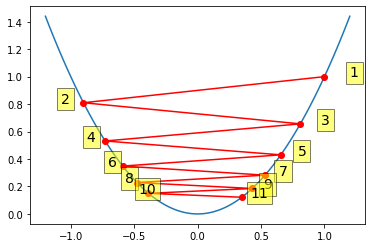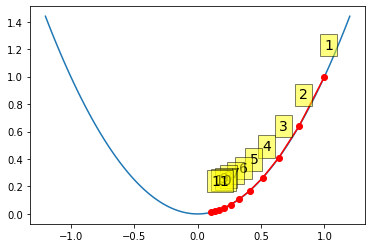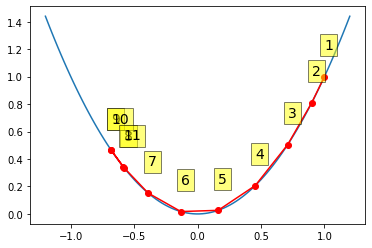# DS 4420: Backprop¶

In :
import torch
from torch import nn


### Revisiting our toy classification network (with a non-linearity)¶

In :
class ToyMLP(nn.Module):

def __init__(self):
super(ToyMLP,self).__init__()
self.i = nn.Linear(3, 2, bias=False)
self.a = nn.Sigmoid()
self.o = nn.Linear(2, 1, bias=False)

def forward(self, X):
# Layer 1
z_h = self.i(X)
h = self.a(z_h)

# Layer 2
z_o = self.o(h)
y_hat = self.a(z_o)
return y_hat

m = ToyMLP()

In :
X_1 = torch.tensor([1, 2, .5])
y_1 = torch.tensor().float()

In :
y_hat_1 = m(X_1) # prediction (forward pass)


#### Let's calculate the gradients "manually"¶

First, for top layer -- as per lecture notes

In :
y = y_1.cpu().detach().numpy()
y_hat = y_hat_1.cpu().detach().numpy()

z_h = m.i(X_1) # raw scores
h = m.a(z_h).cpu().detach().numpy() # hidden, post-activation

# output weights
delta_o = (y_hat - y)
print("delta_o")
print(delta_o)
dL_dWo = h * delta_o  # note that y_hat = \sigma(W2 * h)
print("dL/dW2: {0}".format(dL_dWo))

delta_o
[0.34093398]
dL/dW2: [0.24099095 0.21946827]


This is for $W^{(o)}$, but then how about $W^{(i)}$?

In :
h.shape

Out:
(2,)
In :
X = X_1.cpu().detach().numpy()
Wo = m.o.weight.cpu().detach().numpy().squeeze() # weights
Wi = m.i.weight.cpu().detach().numpy().squeeze() # weights

# h = sigma(z^h)
delta_h = (h * (1 - h) * delta_o) * Wo

In :
print("dL/dW1_1: {0}".format(delta_h * X)) # gradients for weights associated with h_1 node
print("dL/dW1_2: {0}".format(delta_h * X)) # and to those for h_2

dL/dW1_1: [-0.03111681 -0.06223362 -0.0155584 ]
dL/dW1_2: [-0.0422445  -0.084489   -0.02112225]


#### Of course, torch will do this for us!¶

In :
loss_func = torch.nn.BCELoss()
loss = loss_func(y_hat_1, y_1) # incur loss
print(loss)

tensor(0.4169, grad_fn=<BinaryCrossEntropyBackward>)

In :
list(m.o.parameters()).grad is None

Out:
True

In :
loss.backward(retain_graph=True) # backwards pass

In :
list(m.o.parameters()).grad

Out:
tensor([[0.2410, 0.2195]])
In :
list(m.i.parameters()).grad

Out:
tensor([[-0.0311, -0.0622, -0.0156],
[-0.0422, -0.0845, -0.0211]])

## Optimizing via backprop¶

OK but so why were we so concerned with these gradients in the first place again?!?

Right for optimization, i.e., parameter estimation. An important note: The specific method we use for estimation (e.g., vanilla SGD, as we've seen, or more advanced variants) is independent of how we derive the gradients. 'Backprop' is often conflated -- wrongly -- with estimation.

Modern kits provide lots of options for optimizers. Now mindful of this, let's review a basic example in torch.

In :
from torch import optim
m = ToyMLP().cuda()
# parameter vals of first level weights after initialization.
print(list(m.i.parameters()).data)

X_1 = torch.tensor([1, 2, .5]).cuda()
y = torch.tensor().float().cuda()

loss_func = torch.nn.BCELoss()
loss = loss_func(m(X_1), y)
optimizer = optim.SGD(m.parameters(), lr=1)

# 1. calculate gradients (auto-magically via backprop)
# 2. store in parameter objects.
loss.backward()

# the optimizer now uses the calculated gradients to update
# parameter estimates.
optimizer.step()

print("-- after step --")
print(list(m.i.parameters()).data)

tensor([[ 0.3103,  0.1507, -0.0837],
[ 0.4570, -0.4705,  0.1860]], device='cuda:0')
-- after step --
tensor([[ 0.3674,  0.2648, -0.0552],
[ 0.4885, -0.4074,  0.2018]], device='cuda:0')


This is a classic dataset of handwritten digits.In :
import numpy as np
import keras
from keras.datasets import mnist
(X_train, y_train), (X_test, y_test) = mnist.load_data()

# normalize
z = 255 # these are pixel values (0,255)
X_train = X_train / z
X_test  = X_test  / z

set(y_train)

Out:
{0, 1, 2, 3, 4, 5, 6, 7, 8, 9}
In :
y_train = keras.utils.to_categorical(y_train)
y_test  = keras.utils.to_categorical(y_test)

y_train

Out:
array([0., 0., 0., 0., 0., 1., 0., 0., 0., 0.], dtype=float32)
In :
y_test.shape

Out:
(10000, 10)
In :
X_train.shape

Out:
(60000, 28, 28)

Recall that these are 28x28 images. Let's flatten into $28^2$ dimensional vectors and then build a simple feedforward network to classify the digits. Again this is a terrible idea since we are discarding structure -- soon we'll have better models for this.

In :
n = X_train.shape
X_train = X_train.reshape((-1, 28**2))
X_test = X_test.reshape((-1, 28**2))
X_train.shape

Out:
(60000, 784)

This is the same as in the notebook from last lecture, but hopefully what is going on here is more clear now, in particular the backward() function is taking a backward pass through the network and depositing the gradients w.r.t. the loss on each Variable, as we reviewed in lecture for smaller networks. Once these gradients are available, we "step" the optimizer, which uses the gradients to update model parameters.

In :
from torch import optim
def train(m, X, y, epochs=100):
optimizer = optim.SGD(m.parameters(), lr=1)
loss_func = torch.nn.CrossEntropyLoss()

X = torch.from_numpy(X).float()#.cuda()

# CrossEntropyLoss does not expect one-hot encodings, but rather
# wants indices as the target!
y_indices = np.argmax(y, axis=1)
y = torch.from_numpy(y_indices).long()#.cuda()

for epoch in range(epochs):
# zero out the gradients stored on the vars from last
# backwards pass.

# note that we are making all 60k predictions here;
# this is batch GD.
y_hat = m(X)

# and incurring loss for all of them
loss = loss_func(y_hat, y)
loss.backward()

optimizer.step()

if epoch % 100 == 0:
print(epoch, loss.item())

In :
y_test.shape

Out:
(10000, 10)
In :
y_test_indices = np.argmax(y_test, axis=1)

In :
class MLP(nn.Module):

def __init__(self):
super(MLP,self).__init__()
self.i = nn.Linear(28**2, 32)
self.a = nn.Sigmoid()
self.o = nn.Linear(32, 10)
self.sm = nn.Softmax()

def forward(self, X):
h = self.i(X)
h = self.a(h)
y_hat = self.o(h)
y_hat = self.sm(y_hat)
return y_hat

m = MLP()#.cuda()

In :
train(m, X_train, y_train, epochs=1000)

/anaconda3/lib/python3.7/site-packages/ipykernel_launcher.py:14: UserWarning: Implicit dimension choice for softmax has been deprecated. Change the call to include dim=X as an argument.


0 2.302537441253662
100 2.1512205600738525
200 1.9821455478668213
300 1.896279215812683
400 1.8432165384292603
500 1.8035039901733398
600 1.7513976097106934
700 1.7306667566299438
800 1.720908522605896
900 1.7148447036743164

In :
y_hat_test = m(torch.from_numpy(X_test).float())

/anaconda3/lib/python3.7/site-packages/ipykernel_launcher.py:14: UserWarning: Implicit dimension choice for softmax has been deprecated. Change the call to include dim=X as an argument.


In :
y_hat_test = np.argmax(y_hat_test.detach().numpy(), axis=1)

In :
import sklearn
from sklearn.metrics import accuracy_score
accuracy_score(y_hat_test, y_test_indices)

Out:
0.7647

### Vanilla GD has some pathologies¶

#### Oscillation due to step size¶

Credit for this pretty example to: Cliburn Chan (http://people.duke.edu/~ccc14/sta-663-2018/).

In :
import numpy as np
import matplotlib.pyplot as plt

In :
def f(x):
return x**2

return 2*x

xs = np.zeros(1 + max_iter)
xs = x
for i in range(max_iter):
x = x - alpha * grad(x)
xs[i+1] = x
return xs

In :
alpha = 0.95 # relatively large stepsize
xp = np.linspace(-1.2, 1.2, 100)
plt.plot(xp, f(xp))
plt.plot(xs, f(xs), 'o-', c='red')
for i, (x, y) in enumerate(zip(xs, f(xs)), 1):
plt.text(x*1.2, y, i,
bbox=dict(facecolor='yellow', alpha=0.5), fontsize=14)In :
alpha = 0.1 # small step size
x0 = 1
xp = np.linspace(-1.2, 1.2, 100)
plt.plot(xp, f(xp))
plt.plot(xs, f(xs), 'o-', c='red')
for i, (x, y) in enumerate(zip(xs, f(xs)), 1):
plt.text(x, y+0.2, i,
bbox=dict(facecolor='yellow', alpha=0.5), fontsize=14)Momentum, where we sort of keep in mind the history of the steps taken thus far (via an exponential moving average), can also sometimes help.

In :
def gd_momentum(x, grad, alpha, beta=0.9, max_iter=10):
xs = np.zeros(1 + max_iter)
xs = x
v = 0
for i in range(max_iter):
vc = v/(1+beta**(i+1))
x = x - alpha * vc
xs[i+1] = x
return xs

In :
alpha = 0.95
xs = gd_momentum(1, grad, alpha, beta=0.9)
xp = np.linspace(-1.2, 1.2, 100)
plt.plot(xp, f(xp))
plt.plot(xs, f(xs), 'o-', c='red')
for i, (x, y) in enumerate(zip(xs, f(xs)), 1):
plt.text(x, y+0.2, i,
bbox=dict(facecolor='yellow', alpha=0.5), fontsize=14)In :